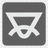The Angles of a Triangle
Elementary+
English FR JA RU UK

You are given the lengths for each side on a triangle. You need to find all three angles for this triangle. If the given side lengths cannot form a triangle (or form a degenerated triangle), then you must return all angles as 0 (zero). .... Each angle is measured in degrees and rounded to the nearest integer number (Standard mathematical rounding).

You should be an authorized user in order to see the full description and start solving this mission.